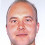# Learning Clojure

## Thursday, September 19, 2013

### Putting it all together : Using Union-Find in Kruskal's Algorithm

```;; Kruskal's Algorithm and Union-Find

;; So I want to try out my version of union-find on the inspiration for it, Kruskal's algorithm

;; Here's my attempt:

(defn make-union-find [elts]
(apply hash-map (mapcat (fn[x][x [x [x]]]) elts)))

(defn joined? [union-find a b]
(= (first (union-find a)) (first (union-find b))))

(defn join [union-find a b]
union-find ;; nothing to do
(if (>= (count followers-a) (count followers-b))  ;; if a's in the bigger group
(let [new-followers (into followers-a followers-b)] ;; combine follower-lists
(reduce (fn[uf x] (assoc uf x [leader-a []])) uf followers-b))) ;; and change every member of the 'b group' to follow the 'a group leader' instead
(join union-find b a)))))) ;; but if a's in the smaller group do it the other way round

;; And here's the data from the original maximum-spanning-tree problem

(def cities ["London" "Birmingham" "Sheffield" "Bristol" "Leeds" "Liverpool" "Manchester"])

[
["London" "Birmingham" 103]
["London" "Sheffield"  167]
["London" "Leeds" 175]
["London" "Bristol" 100]
["London" "Liverpool" 178]
["London" "Manchester" 181]

["Birmingham" "Sheffield"  91]
["Birmingham" "Leeds" 92 ]
["Birmingham" "Bristol" 79 ]
["Birmingham" "Liverpool" 75 ]
["Birmingham" "Manchester" 95]

["Sheffield" "Bristol" 180]
["Sheffield" "Leeds" 33]
["Sheffield" "Liverpool" 63]
["Sheffield" "Manchester" 37]

["Bristol" "Leeds" 171]
["Bristol" "Liverpool" 136]
["Bristol" "Manchester" 139]

["Leeds" "Liverpool" 73]
["Leeds" "Manchester" 40]

["Liverpool" "Manchester" 27]])

;; Kruskal's Algorithm Again:

;; Order the links by cost

;; And construct an initial partition of the cities
(def city-uf (make-union-find cities))

;; Kruskal has told us that should go through our list of links in cost order, building

;; This function takes the partition so far, and the current partially constructed spanning tree
;; and adds a new link to the tree it is building
;; if and only if the link helps
(if (joined? uf a b)
[uf tree]
[(join uf a b) (cons link tree)])))

;; By the Power of Kruskal and by that of Union-Find:

;; Well, they are surely the same answers as before.

(time (reduce add-link [(make-union-find (range 0 10)) '()] (take 10 (repeatedly (fn[] (list (rand-int 10) (rand-int 10) (rand-int 10)))))))
"Elapsed time: 3.388981 msecs"
(time (reduce add-link [(make-union-find (range 0 100)) '()] (take 100 (repeatedly (fn[] (list (rand-int 100) (rand-int 100) (rand-int 100)))))))
"Elapsed time: 15.278055 msecs"
(time (reduce add-link [(make-union-find (range 0 1000)) '()] (take 1000 (repeatedly (fn[] (list (rand-int 1000) (rand-int 1000) (rand-int 1000)))))))
"Elapsed time: 96.710761 msecs"
(time (reduce add-link [(make-union-find (range 0 10000)) '()] (take 10000 (repeatedly (fn[] (list (rand-int 10000) (rand-int 10000) (rand-int 10000)))))))
"Elapsed time: 978.454873 msecs"
(time (reduce add-link [(make-union-find (range 0 100000)) '()] (take 100000 (repeatedly (fn[] (list (rand-int 100000) (rand-int 100000) (rand-int 100000)))))))
"Elapsed time: 15033.289079 msecs"

;; So far we're looking pretty linear, as I was expecting, but suddenly:

(time (reduce add-link [(make-union-find (range 0 200000)) '()] (take 200000 (repeatedly (fn[] (list (rand-int 200000) (rand-int 200000) (rand-int 200000)))))))
"Elapsed time: 129027.687251 msecs"
(time (reduce add-link [(make-union-find (range 0 1000000)) '()] (take 1000000 (repeatedly (fn[] (list (rand-int 1000000) (rand-int 1000000) (rand-int 1000000)))))))
"Elapsed time: 500747.749151 msecs"

;; Not sure what happened there! Anyone know?
```

1.Thanks for sharing your code !
Did you know about https://github.com/jordanlewis/data.union-find ?

Cheers,
B.

1.B. Thanks!

Actually I did know about it (in fact I've linked to it in a previous post) but I haven't tried it yet. I want to understand how these things work and the best way is to write one yourself. I intend to make further posts about the lazy-union strategy, if I don't get distracted. I can't imagine anyone's going to be reading them, but I like writing them and it helps get the ideas clear in my mind if I try to explain them to an imaginary readership.